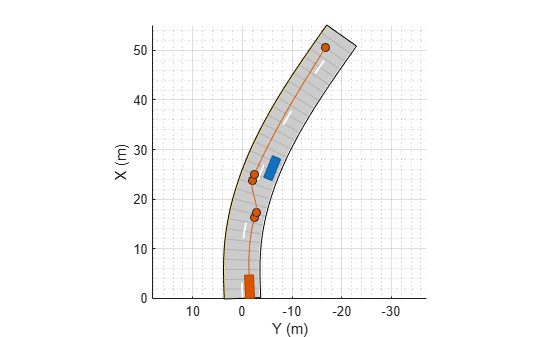# record

Run driving scenario and record actor states

## Syntax

``rec = record(scenario)``

## Description

example

````rec = record(scenario)` returns a recording, `rec`, of the states of actors in a driving scenario simulation, `scenario`. To record a scenario, you must define the trajectory of at least one actor.```

## Examples

collapse all

Create a driving scenario in which one car passes a stationary car on a two-lane road.

```scenario = drivingScenario; road(scenario,[0 0; 10 0; 53 -20],'lanes',lanespec(2)); plot(scenario,'Waypoints','on'); stationaryCar = vehicle(scenario,'Position',[25 -5.5 0],'Yaw',-22); passingCar = vehicle(scenario); waypoints = [1 -1.5; 16.36 -2.5; 17.35 -2.765; ... 23.83 -2.01; 24.9 -2.4; 50.5 -16.7]; speed = 15; % m/s trajectory(passingCar,waypoints,speed);```Record the driving scenario simulation.

`rec = record(scenario);`Compare the recorded poses of the passing car at the start and end of the simulation.

`rec(1).ActorPoses(2)`
```ans = struct with fields: ActorID: 2 Position: [1 -1.5000 0] Velocity: [14.9816 0.7423 0] Roll: 0 Pitch: 0 Yaw: 2.8367 AngularVelocity: [0 0 1.2537e-05] ```
`rec(end).ActorPoses(2)`
```ans = struct with fields: ActorID: 2 Position: [50.4717 -16.6823 0] Velocity: [12.7171 -7.9546 0] Roll: 0 Pitch: 0 Yaw: -32.0261 AngularVelocity: [0 0 -0.0099] ```

## Input Arguments

collapse all

Driving scenario, specified as a `drivingScenario` object.

## Output Arguments

collapse all

Recording of actor states during simulation, returned as an M-by-1 vector of structures. M is the number of time steps in the simulation. Each structure corresponds to a simulation time step.

The `rec` structure has these fields:

FieldDescriptionType
`SimulationTime`Simulation time at each time stepReal scalar
`ActorPoses`Actor poses in scenario coordinatesN-by-1 vector of `ActorPoses` structures, where N is the number of actors, including vehicles.

Each `ActorPoses` structure has these fields.

FieldDescription
`ActorID`

Scenario-defined actor identifier, specified as a positive integer.

`Position`

Position of actor, specified as a real-valued vector of the form [x y z]. Units are in meters.

`Velocity`

Velocity (v) of actor in the x-, y-, and z-direction, specified as a real-valued vector of the form [vx vy vz]. Units are in meters per second.

`Roll`

Roll angle of actor, specified as a real scalar. Units are in degrees.

`Pitch`

Pitch angle of actor, specified as a real scalar. Units are in degrees.

`Yaw`

Yaw angle of actor, specified as a real scalar. Units are in degrees.

`AngularVelocity`

Angular velocity (ω) of actor in the x-, y-, and z-direction, specified as a real-valued vector of the form [ωx ωy ωz]. Units are in degrees per second.

For full definitions of these structure fields, see the `actor` and `vehicle` functions.

Data Types: `struct`